# Algebra 2/Trigonometry

Welcome to Algebra 2/Trigonometry! Below you will find a list of lessons, videos, and practice questions all relating to Algebra 2/Trigonometry. If you’re looking to make math suck just a little bit less, think about Subscribing to our Youtube channel for the latest MathSux videos updated every week! Thanks for stopping by and happy calculating!Expand a Cubed BinomialSynthetic Division & Factoring PolynomialsLog EquationsFactor by Grouping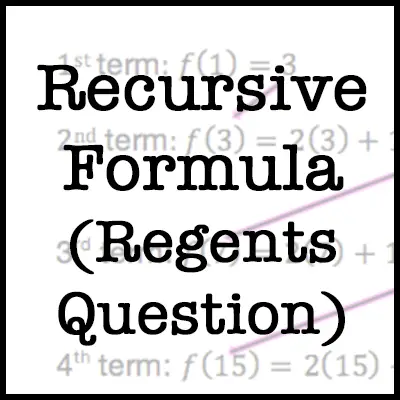Recursive Formula (Regents Question)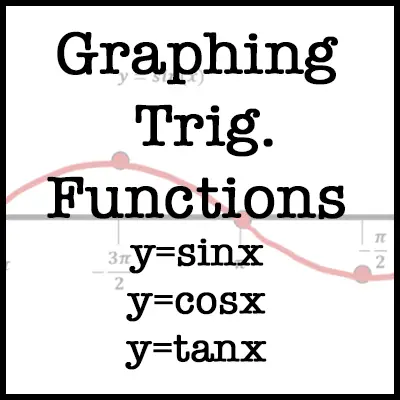Graphing Trig. Functions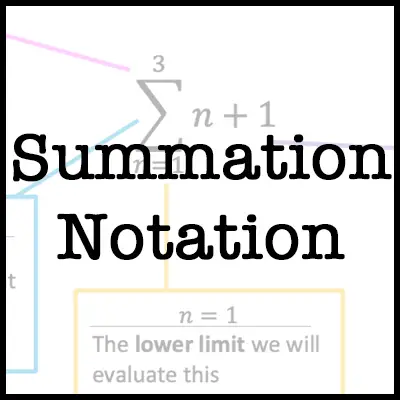Summation NotationExpand a Cubed Binomial (Regents)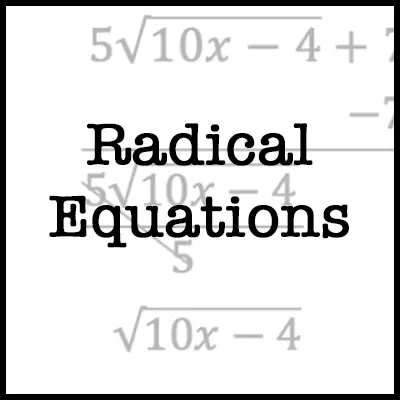Solving Radical Equations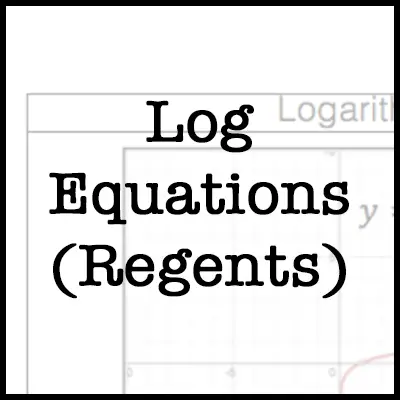Log Equations (Regents)Imaginary Numbers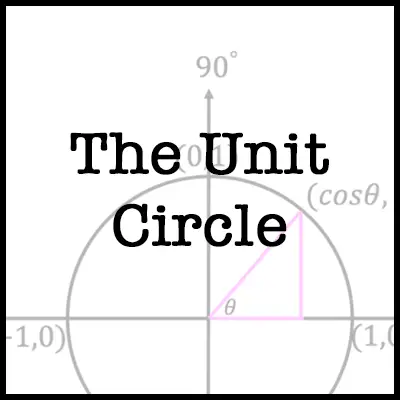The Unit CircleGraphing Trig. FunctionsFactor by Grouping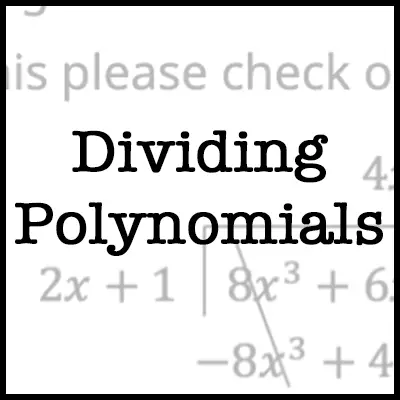Dividing Polynomials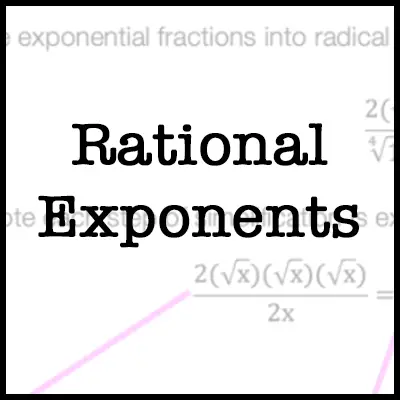Rational ExponentsFactoring Trinomials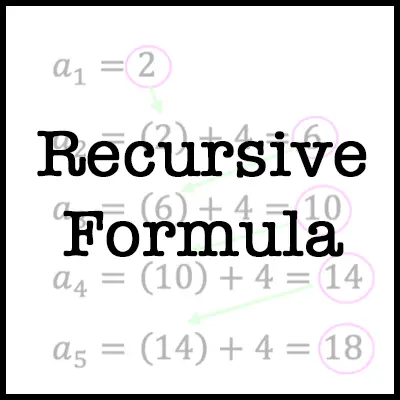Recursive FormulaSolving Trigonometric EquationsCompound Interest Formula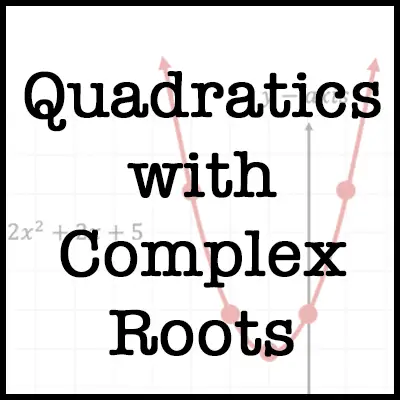Quadratics with complex Roots

Looking for more lessons? Don’t forget to check out Statistics here!